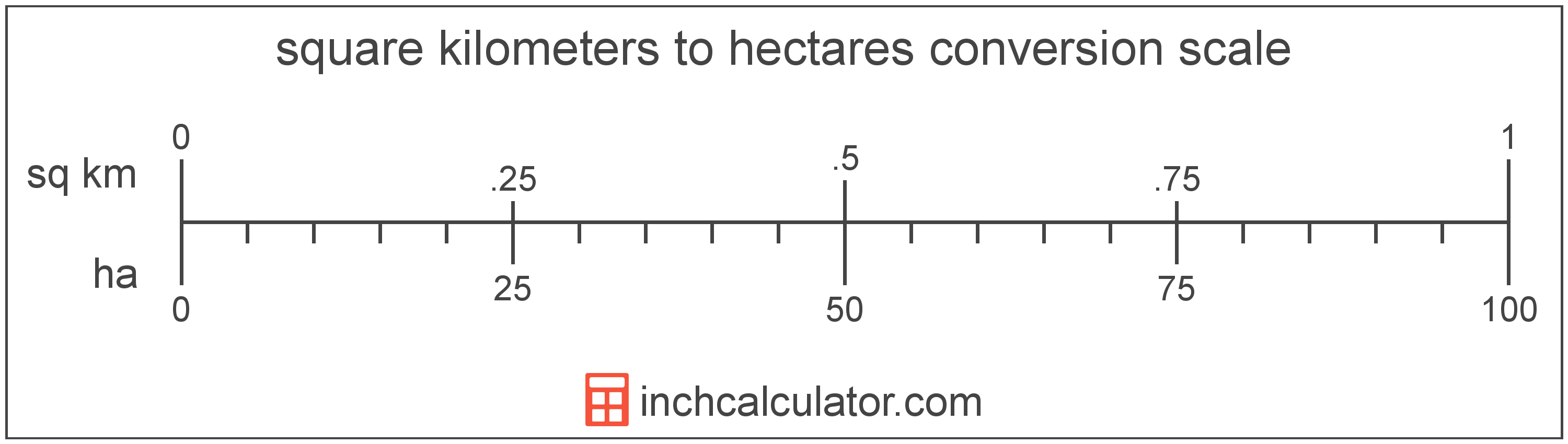# Hectares to Square Kilometers Converter

Enter the area in hectares below to get the value converted to square kilometers.

Results in Square Kilometers:1 ha = 0.01 sq km

Do you want to convert square kilometers to hectares?

## How to Convert Hectares to Square Kilometers

To convert a measurement in hectares to a measurement in square kilometers, divide the area by the following conversion ratio: 100 hectares/square kilometer.

Since one square kilometer is equal to 100 hectares, you can use this simple formula to convert:

square kilometers = hectares ÷ 100

The area in square kilometers is equal to the area in hectares divided by 100.

For example, here's how to convert 5 hectares to square kilometers using the formula above.
square kilometers = (5 ha ÷ 100) = 0.05 sq km## What Is a Hectare?

One hectare is equal to 10,000 square meters, or the area of a square with 100 meter sides.

The hectare is an SI accepted unit for area for use with the metric system. In the metric system, "hecto" is the prefix for 102. Hectares can be abbreviated as ha; for example, 1 hectare can be written as 1 ha.

## What Is a Square Kilometer?

One square kilometer is equivalent to the area of a square with sides that are each 1 kilometer in length. One square kilometer is equal to 0.386102 square miles or 1,000,000 square meters.

The square kilometer, or square kilometre, is a multiple of the square meter, which is the SI derived unit for area. In the metric system, "kilo" is the prefix for thousands, or 103. A square kilometer is sometimes also referred to as a square km. Square kilometers can be abbreviated as sq km, and are also sometimes abbreviated as km². For example, 1 square kilometer can be written as 1 sq km or 1 km².

## Hectare to Square Kilometer Conversion Table

Table showing various hectare measurements converted to square kilometers.
Hectares Square Kilometers
1 ha 0.01 sq km
2 ha 0.02 sq km
3 ha 0.03 sq km
4 ha 0.04 sq km
5 ha 0.05 sq km
6 ha 0.06 sq km
7 ha 0.07 sq km
8 ha 0.08 sq km
9 ha 0.09 sq km
10 ha 0.1 sq km
11 ha 0.11 sq km
12 ha 0.12 sq km
13 ha 0.13 sq km
14 ha 0.14 sq km
15 ha 0.15 sq km
16 ha 0.16 sq km
17 ha 0.17 sq km
18 ha 0.18 sq km
19 ha 0.19 sq km
20 ha 0.2 sq km
21 ha 0.21 sq km
22 ha 0.22 sq km
23 ha 0.23 sq km
24 ha 0.24 sq km
25 ha 0.25 sq km
26 ha 0.26 sq km
27 ha 0.27 sq km
28 ha 0.28 sq km
29 ha 0.29 sq km
30 ha 0.3 sq km
31 ha 0.31 sq km
32 ha 0.32 sq km
33 ha 0.33 sq km
34 ha 0.34 sq km
35 ha 0.35 sq km
36 ha 0.36 sq km
37 ha 0.37 sq km
38 ha 0.38 sq km
39 ha 0.39 sq km
40 ha 0.4 sq km

## References

1. Cambridge Dictionary, hectare, https://dictionary.cambridge.org/us/dictionary/english/hectare